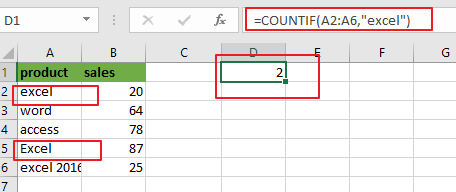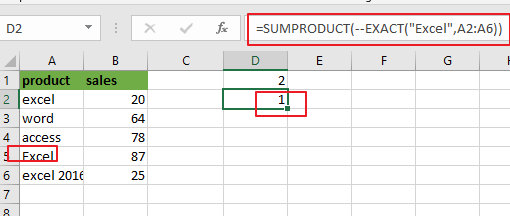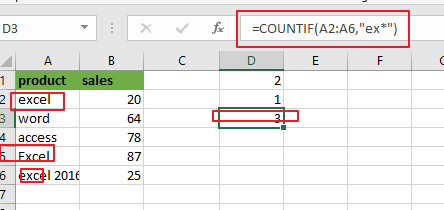# How to Count Cells equals to a Specific Value in Excel

This post will guide you how to count the number of cells equal to a specific value in a given range cells using a formula in Excel 2013/2016. How do I count the cells which are exactly equal to a numeric value or a text string in Excel. Is there a easy way to count cells partial equal to a cell values in a selected range of cells in Excel.

Assuming that you want to count cells that contain value defined in a selected range of cells. In this case, you can use the COUNTIF function to count cells in a range cells that exactly match a given criteria. Or you can use the SUMPRODUCT function combining with the EXACT function to count cells exactly equal to with case sensitive in your worksheet.

## Count Cells Exactly Equal to a Value

To Count the number of cells in range A2:A6 that equal to “excel” without case sensitive, you can use the below formula based on the COUNTIF function:

=COUNTIF(A2:A6,”excel”)Let’s see how this formula works:

The COUNTIF function can be used to count the number of cells in a range that match the supplied criteria in Microsoft Excel. The range A2:A6 is the range of cells in which you want to match the criteria. The criteria “excel” is the reference of values that you want to count in the range “A2:A6”.

The COUNTIF function is not case-sensitive. So if you wish to count cells exactly equal to a specific value, and you should use “EXACT” function. Using the below formula to count the number of cells in range A2:A6 that match the criteria with case sensitive.

=SUMPRODUCT(–EXACT(“Excel”,A2:A6))## Count Cells Partial Equal to a Value

If you wish to count the number of cells that partially match the supplied criteria. You can use the wildcard in the criteria. Assume that you want to count cells in rang A2:A6 that partially match “el*” text string, just use the following COUNTIF function:

=COUNTIF(A2:A6,”ex*”)

You can type this formula into a blank cell and press Enter key to get the result.## Count cells Equal to one or Many Cells

If you want to count cells that contain value define in other cells. And in this case, you just only use the COUNTIF function will not work. And you should use another formula based on the SUMPRODUCT function combining with the COUNTIF function to achieve the result.

For example, you want to count the number of the total of “excel” and “word” in range A2:A6, you can use the below generic formula to get the total count of each value:

=SUMPRODUCT(COUNTIF(A2:A6,C2:C3))

It returns 3. The “excel” is found 2 times, and the “word” text string is found 1 time.

Let’s see how this formula works:

The COUNTIF formula will be as an array formula, and return the count of the first value. And it will return an array of counts for each specific value in range C2:C3, such as: {2,1} is the returned array result. This array result will be passed to the SUMPRODUCT function as its argument. And it will sums up the counts and get the count of each value in a range.### Related Functions

• Excel SUMPRODUCT function
The Excel SUMPRODUCT function multiplies corresponding components in the given one or more arrays or ranges, and returns the sum of those products.The syntax of the SUMPRODUCT function is as below:= SUMPRODUCT (array1,[array2],…)…
• Excel COUNTIF function
The Excel COUNTIF function will count the number of cells in a range that meet a given criteria. This function can be used to count the different kinds of cells with number, date, text values, blank, non-blanks, or containing specific characters.etc.= COUNTIF (range, criteria)…
• Excel EXACT function
The Excel EXACT function compares if two text strings are the same and returns TRUE if they are the same, Or, it will return FALSE.The syntax of the EXACT function is as below:= EXACT (text1,text2)…